# Investigation of Thermal Collector Nanofluids to Increase the Efficiency of Photovoltaic Solar Cells

Investigation of Thermal Collector Nanofluids to Increase the Efficiency of Photovoltaic Solar Cells
Department of Mechanical Engineering, Faculty of Engineering, Universitas Sebelas Maret, Surakarta 57126, Indonesia

Corresponding Author Email:
zainal_arifin@staff.uns.ac.id
Page:
415-422
|
DOI:
https://doi.org/10.18280/ijht.400208
2 February 2022
|
Accepted:
6 April 2022
|
Published:
30 April 2022
| Citation

OPEN ACCESS

Abstract:

Active cooling technology of photovoltaic thermal (PVT) system allows lowering the working temperature to increase the efficiency of photovoltaic (PV) solar cells. In addition, the absorbed heat produces thermal energy simultaneously through the working fluid in the collector. Therefore, in this study, the modeling of the riser configuration of the PVT collector for cooling PV solar cells was carried out using a working fluid and varied mass flow rates. It is used to find the effect of working fluid and mass flow rate on the resulting efficiency. ANSYS 18.2 system analysis software is used to simulate phenomena that occur. The natural convection phenomena of the PV cell layer were explored using ANSYS Steady State-Thermal. The simulation of the heat transfer phenomenon between the PV cell layer and the liquid was done using ANSYS Fluent. The improvement in energy generation efficiency achieved by utilizing a thermal collector system to cool PV solar cells reaches 49.8%. The maximum PV solar cell electrical efficiency value comes when the rectangular collector riser arrangement is modeled with SiO2 nanofluid working fluid and a mass flow rate of 0.01 kg/s. Each variation used was not significantly different from the efficiency of the PV solar cells produced.

Keywords:

PV, thermal collector, nanofluids, ANSYS

1. Introduction

PV Thermal Collector System (PVT) is a photovoltaic solar cell active cooling technique. Installing a collector underneath the solar cell accomplishes this strategy. As a result, while it generates energy, it is cooled by the heat transfer medium in the collector. PVT systems simultaneously generate electricity and heat. Air PVT and water PVT are two of the most prevalent cooling techniques for photovoltaic solar cells. Refrigerants, water, nanofluids, air, and a mix of liquid and air can all be used for liquid cooling. The advantages of air cooling include increased overall efficiency, no financial limits, and hot air that may be used to heat buildings in the winter. However, the resulting efficiency is lower than water cooling [1, 2].

PVT is built with a different way to disperse heat on the PV surface, resulting in more electrical energy. Much research has been conducted to investigate the elements that influence PVT performance in simulations or experiments. Design parameters, operating parameters, and climate parameters are all variables that can impact performance [3, 4]. Various designs have been examined to improve photoelectric performance, and PVT collectors have been presented as a solution. A high thermal conductivity between the heat absorption unit and the solar module can improve both electrical and thermal efficiency .

The critical functional part is the PVT solar cell collector, which transmits the photovoltaic laminate that has collected sun-induced heat transfer to the fluid. The heat from the solar cell must be able to travel through the fluid due to the flow design . The straight flow design has been claimed to be the best flow design. A direct flow pipe has a higher efficiency of 64% than a single-pass pipe . The collector's specific heat capacity should be below to respond quickly to changing climatic conditions (such as in the case of fluctuating conditions) and to optimize the management of the available thermal energy, even in small quantities. Thermal conductivity and specific heat capacity are the two most important properties. PVT collectors usually use metals such as copper, aluminum, or stainless steel . Experiments were carried out on the sort of aluminum pipe used by the researchers. When water or nanofluids pass through aluminum pipes, the effect is that they are not eroded. The collector's top provides optimal adherence to the PV cell or laminate, enhancing heat dissipation of the photovoltaic components [9, 10]. The forming process used to make different types of plates influences the material used and the channel design. The contact area between the collector surface and the PV affects heat transmission [11, 12].

Nanofluids are suspensions of nanoparticles in fluids that significantly enhance their properties at modest nanoparticle concentrations [13, 14]. Decade research on nanofluids has solidified the claim that they have excellent heat transfer properties. Nanofluids have been widely used in various fields because of their unique thermal conductivity [15, 16]. In addition to their application in solar collector thermal collectors, nanofluids are also used in photoelectric systems. In order to better convert solar energy into electric energy, the photoelectric photovoltaic system must keep the battery's temperature at a specific temperature because the conversion efficiency will be too low if the battery temperature of the battery is too high .

The CFD (Computational Fluid Dynamic) approach may be used to estimate the performance characteristics of the PVT solar collector based on an examination of the riser arrangement on the PVT collector for cooling PV solar cells using a working fluid varying mass flow rates . The working fluid is an essential part of PVT systems. Air, water, and refrigerants are common working fluids used as a coolant in PVT systems . Therefore, people began to add nanofluids to the PVT collector to absorb waste heat and fully use its vitality. Compared to other cooling technologies, nanofluid cooling improves thermal performance and improves total power generation [19, 20].

CFD is a branch of fluid dynamics that solves and analyzes issues with a fluid flow connected to the distribution of temperature and pressure. CFD aims to make it easier to grasp fluid flow characteristics by displaying the findings in Figures, vectors, contours, and 3D animations [6, 21]. From this basis, it is necessary to simulate the collector performance using the CFD method with ANSYS software to obtain its performance characteristics [22, 23].

Given the progress made in the design of photovoltaic thermal collector systems. The main concern in this study is the application of PVT systems to generate more electrical energy. CFD simulations compare the best working fluids used to conduct heat on the panel. The study also analyzed the variance (ANOVA) test to determine the difference in the mean data between treatment variations on the resulting efficiency.

2. Methods

2.1 Material

In this experiment, we used PV with materials and their properties, as shown in Table 1.

2.2 Modeling

The heat flux delivered to the PV solar cell surface influences the research's modeling and simulation, with only convection losses occurring. Fluid flows from the intake to the photovoltaic (PV) solar cell's exit. This procedure reduces temperature and is used to test the efficiency of PV solar cells. Figure 1 (a) depicts a flow diagram of the procedure. The material studied in this study is a 660 x 540 x 4.33 mm photovoltaic solar cell module with a temperature coefficient of -0.4% /K . Table 1 and Figure 1 (b) depict the architecture and parameters of solar cells. While the design of the 15 x 30 mm thermal collector indirect flow refers to the research as shown in Figure 1 (c) .

Table 1. Specifications of layers on PV cells 

 Layers Density(kg/m3) Specific heat capacity(J/kgK) Thermal conductivity(W/mK) Thickness(mm) Glass 2450 790 0.70 3.20 EVA 960 2090 0.31 0.50 PV cell 2330 677 130 0.21 EVA 960 2090 0.31 0.50 PDF 1200 1250 0.15 0.30

## 1.pngFigure 1. (a) PVT model schematic (b) PV (c) Thermal collector (in mm)

2.3 Simulation

ANSYS FLUENT and ANSYS-STEADY STATE THERMAL were used to model the collector system. Figure 2 depicts a simulation in which the heat transfer between the PV solar cell layer and water was modeled using fluent software. In contrast, just the heat transfer from natural convection in the PV cell layer was investigated using steady state thermal software. The state is the simulated steady-state.

## 2.png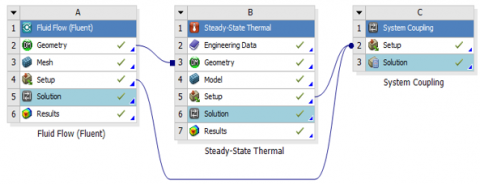Figure 2. ANSYS coupled simulation scheme

For simulation meshing, this work employs a 14 mm element size. The 14 mm mesh element is more stable, allowing for a more accurate recording of flow and heat transfer phenomena in the collector's vicinity. On the PV and collector, the number of elements for the geometry with 14 mm meshes is 12375 and 207746, respectively.

The turbulence model k- the ANSYS FLUENT program uses Renormalization Group (RNG) and steady-state simulation. Fluid mass flow rates of 0.0025, 0.005, 0.0075, and 0.01 kg/s were tested at a temperature of 298.15 K, turbulence intensity of 5%, and hydraulic diameter of 0.02 m. The researchers employed water fluids, CuO, ZnO, and SiO2 nanofluids with the properties listed in Table 2. Coupled Green Gauss Cell-Based is the solution approach employed. The set of convergent criteria is 10-6 for energy equations and 10-4 for pressure, velocity, and continuity equations.

Table 2. Fluid characteristics with 1%wt @298K [27, 28]

 Fluid Type Thermal Conductivity W/mK Density kg/m3 Viscosity mPa/s Specific Heat Capacity J/kgK Water 0.600 998 1.010 4182 CuO 0.612 1012 1.098 2845 ZnO 0.618 1025 1,150 2339 SiO2 0.618 1051 1,243 3032

Meanwhile, simulation using steady-state conditions is employed in the ANSYS STEADY STATE THERMAL program. A heat flux module is used to simulate 800 W/m2 solar radiation. Solar radiation is directed toward the PV cell's surface, whereas convection losses influence the other domain surfaces. With a convection coefficient of 10 W/m2K, little convection heat transfer is expected to occur at the PV cell's surface. The fluid-solid interface is employed on the PV's top surface .

Due to the limitations of the tools in modeling solar radiation, the modeled domains, namely the fluid flowing behind the cells and the boundary conditions where the PV cells or collectors have contact with air, must be adjusted according to the material properties of the PV cells and heat sinks. The fluid flows from the inlet direction and exits towards the outlet to produce thermal fluid. The collector thickness is considered 0 m in the Fluent simulation and is not affected by boundaries other than those specified .

2.4 PV solar cell efficiency analysis

The electrical efficiency value of a photovoltaic (PV) cell is inversely proportional to the significant increase in cell operating temperature during the absorption of solar radiation. The effect of temperature on the electrical efficiency of a PV cell/module can be obtained by using fundamental equations.

Pm = ImVm = (FF) IscVoc    (1)

The formula FF, Isc, Voc, and the subscript m are the maximum power point in the module I-V curve. Both open-circuit voltage and fill factor decrease significantly with temperature (as thermally excited electrons begin to dominate the electrical properties of the semiconductor), while short circuit current increases, but only slightly . Thus, the net effect leads to linear relationships in the form of electrical efficiency expressed as an equation.

$\eta_{e l}=\eta_{\text {ref }}\left[1-\beta_{\text {ref }}\left(T_{c}-T_{\text {ref }}\right)\right]$    (2)

where, $\eta_{\text {ref }}$ denotes the PV solar cell's reference efficiency, $\beta_{\text {ref }}$ denotes the PV solar cell's temperature coefficient, and Tref denotes the PV solar cell's starting reference temperature. For silicon-based PV solar cells, $\eta_{\text {ref }}$ and $\beta_{\text {ref }}$ are 0.12 and 0.0045 /℃ respectively, when Tref is 25℃ . Because of $\eta_{\text {ref }}$, $\beta_{\text {ref }}$, and $T_{\text {ref }}$, CFD simulation is utilized to obtain the final average temperature of PV so that it may compute the amount $\eta_{e l}$ with this equation. While the thermal efficiency may be calculated using the following formula:

$\eta_{t h}=\frac{\text { Useful energy gain }}{\text { total solar irradiance received }}$    (3)

$\eta_{t h}=\frac{m c_{p}\left(T_{o}-T_{i}\right)}{I A}$    (4)

where, m denotes the mass flow rate, cp is the specific heat capacity of the heat transfer fluid, and To denotes the output temperature obtained by the CFD simulation of the fluid. Ti denotes the temperature of the fluid when it is first put into the collector. The intensity of the sunlight is represented by I, while the collector's cross-sectional area is represented by A. The combination of thermal efficiency and electrical efficiency yields the overall efficiency .

$\eta_{\text {total }}=\eta_{e l}+\eta_{t h}$     (5)

The study was conducted to assess homogeneity using ANOVA analysis based on statistical values to be presented. The significance value (α) in this study was assumed to be 0.05. The statistical value used is the characteristic of the fluid, as attached in Table 2. Based on the definition of the problem at hand, formulate a hypothesis. The second hypothesis (H02) regarding the use of nanofluids as working fluids states that the efficiency of working fluids is not significantly different from that of photovoltaic solar cells. The third hypothesis for liquid mass flow (H03) holds that mass flow is not significantly different from photovoltaic solar cell efficiency. The null hypothesis can be accepted if the p-value is greater than the significance value. In addition, if the calculated F value is less than or equal to the table F value, the null hypothesis can be accepted .

3. Results and Discussion

The factors affecting the PV-thermal collector system have been established using CFD modeling and simulation (PVT). For maximizing the performance of photovoltaic (PV) solar cells, design elements such as riser collector arrangement, mass flow rate of fluid, and radiation working fluid are taken into account. ANSYS FLUENT and STEADY STATE THERMAL 18.2 are used to simulate optimization. The results of the optimized simulation are discussed in this chapter. All energy exchanges and PVT efficiency (electric and thermal) are computed after modeling the CFD PVT using the entire ANSYS program for all parameters. The impact of each element may be examined using ANOVA analysis based on these results.

3.1 Thermal collector system-PV with different working fluid

3.1.1 Thermal collector system-PV

The modeling of the PV-thermal collector system by varying the working fluid has been successfully carried out. The fluids used are water, CuO nanofluids, ZnO nanofluids, and SiO2 nanofluids. Nanofluids at 1% wt with a temperature of 298.15 K. Fluids with incompressible properties and adapting to the container can be a heat transfer medium in PV solar cells.

Nanofluids are known to produce lower PV and collector outlet temperatures than water fluids, as shown in Figure 3. The lowest temperatures produced in PV solar cells and collector outlets are 304.96 and 304.964 K, namely the use of SiO2 nanofluid. SiO2 nanofluids are better at reducing temperature than CuO and ZnO nanofluids. This can be attributed to the thermophysical properties such as higher thermal conductivity and density of SiO2 nanofluids than other fluids.

## 3.png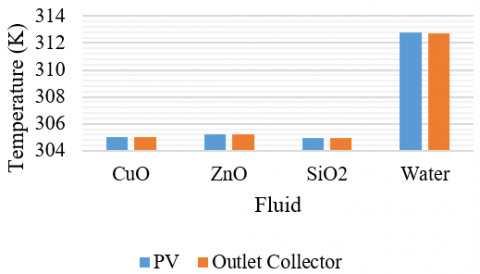Figure 3. The temperature of each working fluid

In Figure 4, nanofluid has fewer color contour lines than the use of water fluid. Based on the resulting temperature distribution contour, SiO2 nanofluid has the lowest range of 298.15 to 304.96 K in PV solar cells and collector outlets. As with thermophysical properties, nanofluids have high thermal conductivity and density values. In general, the temperature will decrease as the density increases. On the contour of the SiO2 nanofluid collector, the color change from green to red is more constant. This is due to the higher viscosity leading to better absorption and heat transfer in PV solar cells.

## 4a.png## 4b.png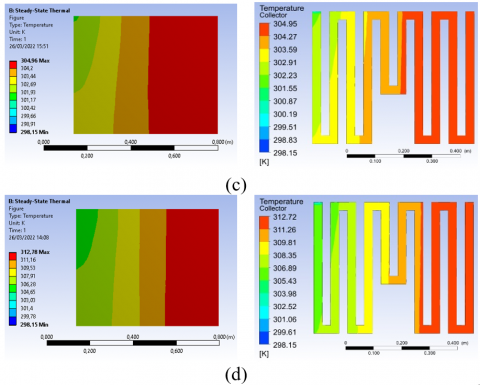Figure 4. Temperature distribution of PV solar cells and collector fluids in the use of working fluids (a) CuO (b) ZnO (c) SiO2 (d) water

3.1.2 Efficiency of thermal collector system-PV

The performance of PV solar cells can be seen from the efficiency of the electricity produced. Nanofluids significantly affect temperature changes in PV solar cells and collector outlets compared to water fluid. So, there is a difference in the resulting electrical and thermal efficiency. The value of electrical efficiency in terms of the difference in temperature produced is compared to the reference temperature. In comparison, the thermal efficiency is strongly influenced by the fluid's specific heat.

## 5.png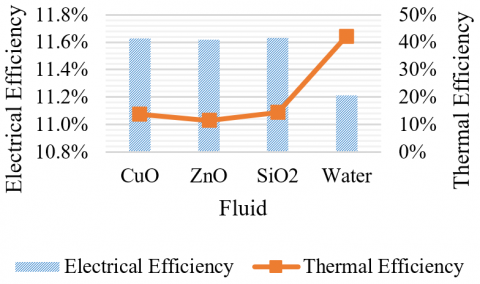Figure 5. PV solar cell efficiency each working fluid

Figure 5 shows that using nanofluids boosts the electrical efficiency of PV solar cells due to the lower temperature of the PV generated compared to water fluid. The usage of SiO2 nanofluids was discovered to be better than the use of CuO and ZnO nanofluids. The electrical efficiency of SiO2 nanofluids is 11.63%, with a thermal efficiency of 14.31%. The relative improvement in the electrical efficiency of PV solar cells grew to 49.80% as the temperature of PV solar cells, and collector output fluid decreased by 19.00%.

3.1.3 Homogeneity value of thermal collector system-PV. efficiency

As illustrated in Figure 5, the usage of fluid types has no discernible effect on the efficiency of the PV solar cells generated. This is also corroborated by the variation value, which is not significantly different from the working fluid data presented in Table 3. As seen in Table 4, it provides a P-value better than 0.05, which is 0.24. This implies that H02 is rejected, as evidenced by the estimated F value, which is less than the F table. As a result, the working fluid consumption does not differ significantly from the electrical efficiency of photovoltaic solar cells.

Table 3. PV solar cell efficiency data each working fluid

 Groups Count Sum Average Variance CuO 3 0.50 0.17 0.01 ZnO 3 0.45 0.15 0.00 SiO2 3 0.53 0.18 0.01 Water 3 1.07 0.36 0.05

Table 4. ANOVA results of PV solar cell efficiency for each working fluid

 Source of Variation SS df MS F P-value F crit Between Groups 0.08 3 0.03 1.74 0.24 4.06 Within Groups 0.13 8 0.02 Total 0.21 11

3.2 Thermal collector system-PV with different fluid mass flow rate

3.2.1 Thermal collector system-PV. temperature distribution

To determine the temperature drop in photovoltaic solar cells, the mass flow rate of the thermal collector system is investigated. The heat transmitted by the fluid flow that passes through the collector impacts the electrical and thermal efficiency . This study used mass flow rates of 0.0025, 0.005, 0.0075, and 0.01 kg/s with a radiation intensity of 800 W/m2. The impact of fluid mass flow rate on temperature is seen in Figure 6. According to the modeling findings, a mass flow rate of 0.01 kg/s resulted in the lowest temperature of 303.64 K. The temperature drops as the mass flow rate of the fluid increases, as seen by the graph's trend.

The simulation results reveal different outlines for each temperature fall. Because the flow path is straight, there are variations in the color contours generated and dispersed in Figure 7. The red contour indicates high temperature, whereas the green contour indicates low temperature. With a mass flow rate of 0.01 kg/s, the fluid displays a more even color contour on the collector and a more dominant red contour on the PV surface. With a temperature range of 298.15 to 303.64 K, it was evident that it has a low-temperature distribution in PV solar cells and collectors. Heat transfer occurs in the PV solar cells, converted better and overall to the collector fluid as the fluid flow velocity rises since the mass flow rate of water is directly proportional to the velocity of the water.

## 6.pngFigure 6. The temperature of each fluid mass flow rate

## 7a.png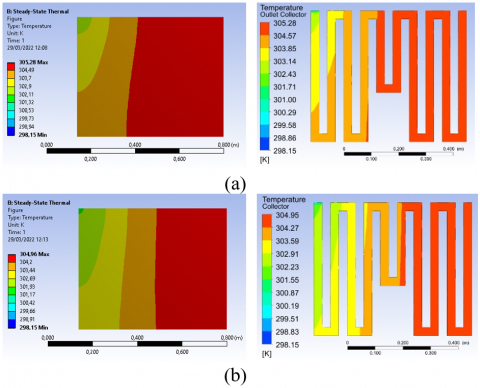## 7b.png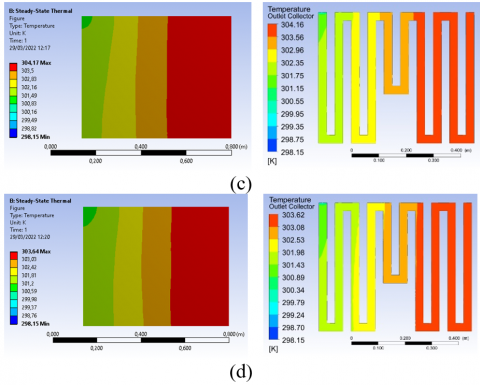Figure 7. Temperature distribution of PV solar cells and collector fluids in the use of fluid mass flow rate (a) 0.0025 (b) 0.005 (c) 0.0075 (d) 0.01 kg/s

3.2.2 Efficiency of thermal collector system-PV

When a collector system is used to cool photovoltaic solar cells, the mass flow of the fluid affects temperature fluctuations. Temperature changes caused by heat transfer from the solar cells to the working fluid provide electrical and thermal energy. The generated electrical and thermal energy can be used to study the performance improvement of photovoltaic solar cells. The electrical efficiency of PV solar cells was estimated by changing the maximum operating temperature of the PV. In contrast, the thermal efficiency was calculated by changing the liquid inlet and outlet temperatures.

The modeling process using a photovoltaic collector system with a radiation intensity of 800 W/m2 resulted in a successful temperature change. There is a significant change in electrical efficiency at the mass flow rates used in Figure 8. Based on the following trends, it is known that the faster the mass flow of the fluid, the more efficient the energy generated. Using a fluid mass flow of 0.01 kg/s, the maximum electrical efficiency is 11.70%, and the thermal efficiency is 15.89%. The high electrical efficiency is due to a 19.39% lower PV maximum temperature than a system without a collector. The PV solar cell temperature decreases when the fluid's mass flow affects the heat transfer that occurs in the PV to the working fluid. Therefore, changing the mass flow of the fluid affects the efficiency of the energy produced . When employing a fluid flow rate of 0.01 kg/s and not using a thermal collector system, the relative difference in electrical efficiency obtained is 50.75%.

## 8.png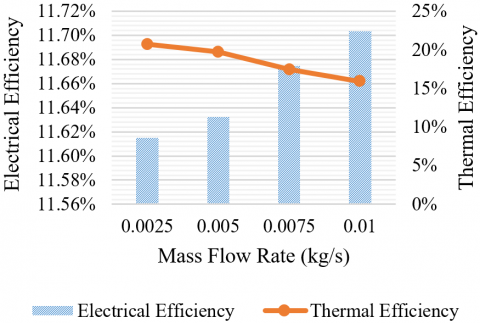Figure 8. PV solar cell efficiency each fluid mass flow rate

3.2.3 Homogeneity value of thermal collector system-PV. efficiency

The effect of the mass flow rate of the fluid on the efficiency of the resulting electricity was further investigated using ANOVA analysis. As in Table 5 regarding electricity efficiency data, there is no difference in the resulting variations. So, it can be calculated that the calculated F value has a smaller value than the F table value of 0.07. While the resulting P-value of 0.97 is greater than the specified significance value, as in Table 6. Based on this, H03 is accepted. So, it can be said that the mass flow rate is not significantly different from the electrical efficiency of photovoltaic solar cells.

Table 5. PV solar cell efficiency data each fluid mass flow rate

 Groups Count Sum Average Variance 0.0025 3 0.65 0.22 0.01 0.0050 3 0.63 0.21 0.01 0.0075 3 0.58 0.19 0.01 0.0100 3 0.55 0.18 0.01

Table 6. ANOVA results of PV solar cell efficiency each fluid mass flow rate

 Source of Variation SS df MS F P-value F crit Between Groups 0.002 3 0.001 0.07 0.98 4.07 Within Groups 0.071 8 0.009 Total 0.073 11
4. Conclusion

Modeling and simulation procedures have effectively studied the usage of thermal collector systems for cooling photovoltaic solar cells. ANSYS Software is used for simulation. Thermal steady-state on photovoltaic solar cells and fluent ANSYS Software on collector fluid. The simulation is linked to calculating temperature changes in the PV solar cells and collector fluid. Temperature changes are used to calculate the performance of photovoltaic solar cells. The increase in electrical efficiency of photovoltaic solar cells with predicted thermal efficiency is used to measure performance. The PV-thermal collector system measures the electrical and thermal efficiency produced by varying the kind of fluid and fluid flow rate.

The addition of nanofluids has proven to increase the efficiency of PVT panels. Based on the simulation results obtained temperature distribution on a more even panel with a small range. This can happen due to the properties of nanofluids that are better at conducting heat. The properties of nanofluids as a working fluid cooling panel can be optimized by varying the mass flow rate in the collector.

SiO2 nanofluid has the best electrical efficiency of 11.63% and the highest thermal efficiency of 14.31%. The PV solar cells and collector output fluid temperature were reduced by 19.0%. As a result, the relative improvement in PV solar cell electrical efficiency reached 49.8%. The working fluid utilized had no discernible effect on photovoltaic solar efficiency. With an F count of 1.74, the resultant homogeneity P-value is 0.24.

The highest photovoltaic solar cell electrical efficiency, 11.70%, is achieved when a fluid mass flow rate of 0.01 kg/s is used. The resulting thermal efficiency is 15.89%. The mass flow rate of the working fluid is not substantially different from the electrical efficiency of photovoltaic solar cells, according to the P-value of 0.97 and the F count of 0.07. The relative difference in temperature changes and the gain in electrical efficiency generated are 19.39% and 50.75%, respectively.

Acknowledgment

The authors thank the Rector of Universitas Sebelas Maret (UNS). This research was fully supported by a PNBP grant from the Universitas Sebelas Maret, Indonesia, titled "Development of a Thermal Collector System with Nano Fluids to Improve Photovoltaic Performance" scheme PUT 2022 Contract Number 254/UN27.22/PT.01.03/2022.

References

 Sheshpoli, A.Z., Jahanian, O., Nikzadfar, K., Delavar, M. (2021). Numerical and experimental investigation on the performance of hybrid PV/thermal systems in the north of Iran. Sol Energy, 215: 108-120. https://doi.org/10.1016/j.solener.2020.12.036

 Huang, G., Wang, K., Curt, S.R., Franchetti, B., Pesmazoglou, I., Markides, C.N. (2020). Performance analysis of fluid-based spectralsplitting hybrid photovoltaic-thermal solar collectors. ECOS 2020 - Proc. 33rd Int. Conf. Effic. Cost, Optim. Simul. Environ. Impact Energy Syst., pp. 1-12.

 Mustapha, M., Fudholi, A., Yen, C.H., Ruslan, M.H., Sopian, K. (2018). Review on energy and exergy analysis of air and water based photovoltaic thermal (PVT) collector. International Journal of Power Electronics and Drive System (IJPEDS), 9(3): 1367-1373. http://doi.org/10.11591/ijpeds.v9.i3.pp1367-1373

 Prasetyo, S.D., Prabowo, A.R., Arifin, Z. (2022). The effect of collector design in increasing PVT performance: Current state and milestone. Materialstoday: Proceedings. https://doi.org/10.1016/j.matpr.2021.12.356

 Widyolar, B., Jiang, L., Ferry, J., Winston, R., Kirk, A., Osowski, M., Cygan, D., Abbasi, H. (2019). Theoretical and experimental performance of a two-stage (50X) hybrid spectrum splitting solar collector tested to 600°C. Applied Energy, 239: 514-525. https://doi.org/10.1016/j.apenergy.2019.01.172

 Rosli, M.A.M., Ping, Y.J., Misha, S., Akop, M.Z., Sopian, K.B., Sohif, M., Al-Shamani, A.N., Saruni, M.A. (2018). Simulation study of computational fluid dynamics on photovoltaic thermal water collector with different designs of absorber tube. Journal of Advanced Research in Fluid Mechanics and Thermal Sciences, 52(1): 12-22.

 Sardouei, M.M., Mortezapour, H., Jafari Naeimi, K. (2018). Temperature distribution and efficiency assessment of different PVT water collector designs. Sādhanā, 43: 84. https://doi.org/10.1007/s12046-018-0826-x

 Prasetyo, S.D., Prabowo, A.R., Arifin, Z. (2022). The effect of collector design in increasing PVT performance: Current state and milestone. Materialstoday Proceedings. https://doi.org/10.1016/j.matpr.2021.12.356

 He, W., Chow, T.T., Ji, J., Lu, J., Pei, G., Chan, L.S. (2006). Hybrid photovoltaic and thermal solar-collector designed for natural circulation of water. Applies Energy, 83(3): 199-210. https://doi.org/10.1016/j.apenergy.2005.02.007

 Arifin, Z., Prasetyo, S.D., Prabowo, A.R., Tjahjana, D.D.D.P., Rachmanto, R.A. (2021). Effect of thermal collector configuration on the photovoltaic heat transfer performance with 3D CFD modeling. Open Engineering, 11(1): 1076-1085. https://doi.org/10.1515/eng-2021-0107

 Baranwal, N.K., Singhal, M.K. (2021). Modeling and simulation of a spiral type hybrid photovoltaic thermal (PV/T) water collector using ANSYS. In: Baredar, P.V., Tangellapalli, S., Solanki, C.S. (eds) Advances in Clean Energy Technologies. Springer Proceedings in Energy. Springer, Singapore. https://doi.org/10.1007/978-981-16-0235-1_10

 Arifin, Z., Tribhuwana, B.A., Kristiawan, B., Tjahjana, D.D.D.P., Hadi, S., Rachmanto, R.A., Prasetyo, S.D., Hijriawan, M. (2022). The effect of soybean wax as a phase change material on the cooling performance of photovoltaic solar panel. International Journal of Heat and Technology, 40(1): 326-332. https://doi.org/10.18280/ijht.400139

 Adun, H., Adedeji, M., Dagbasi, M., Bamisile, O., Senol, M., Kumar, R. (2021). A numerical and exergy analysis of the effect of ternary nanofluid on performance of Photovoltaic thermal collector. Journal of Thermal Analysis and Calorimetry, 145: 1413-1429. https://doi.org/10.1007/s10973-021-10575-y

 Sutanto, B., Arifin, Z., Suyitno, S. (2018). Structural characterisation and optical properties of aluminum-doped zinc oxide nanofibers synthesized by electrospinning. Journal of Engineering Science and Technology, 13(3): 715-724.

 Li, J., Zhang, X., Xu, B., Yuan, M. (2021). Nanofluid research and applications: A review. International Communications in Heat and Mass Transfer, 127: 105543. https://doi.org/10.1016/j.icheatmasstransfer.2021.105543

 Arifin, Z., Soeparman, S., Widhiyanuriyawan, D., Purwanto, A., Dharmanto. (2017). Synthesis, characterisation, and fabrication hollow fibres of Zn-doped TiO2 for dye-sensitized solar cells. Journal of Engineering Science and Technology, 12(5): 1227-1239.

 Xu, P., Zhang, X., Shen, J., Wu, Y., Connelly, K., Yang, T., Tang, T., Xiao, M., Wei, Y., Jiang, K., Chen, C., Xu, P., Wang, H. (2017). A review of thermal absorbers and their integration methods for the combined solar photovoltaic/thermal (PV/T) modules. Renewable and Sustainable Energy Reviews, 75: 839-854. https://doi.org/10.1016/j.rser.2016.11.063

 Qiu, Z., Zhao, X., Li, P., Zhang, X., Ali, S., Tan, J. (2015). Theoretical investigation of the energy performance of a novel MPCM (Microencapsulated Phase Change Material) slurry based PV/T module. Energy, 87: 686-698. https://doi.org/10.1016/j.energy.2015.05.040

 Soltani, S., Kasaeian, A., Sarrafha, H., Wen, D. (2017). An experimental investigation of a hybrid photovoltaic/thermoelectric system with nanofluid application. Solar Energy, 155: 1033-1043. https://doi.org/10.1016/j.solener.2017.06.069

 Said, Z., Arora, S., Bellos, E. (2018). A review on performance and environmental effects of conventional and nanofluid-based thermal photovoltaics. Renewable and Sustainable Energy Reviews, 94: 302-316. https://doi.org/10.1016/j.rser.2018.06.010

 Peng, S., Kon, Y., Watanabe, H. (2022). Effects of sea breeze on urban areas using computation fluid dynamic—a case study of the range of cooling and humidity effects in Sendai, Japan. Sustainability, 14(3): 1074. https://doi.org/10.3390/su14031074

 Ramdani, H., Ould-Lahoucine, C. (2020). Study on the overall energy and exergy performances of a novel water-based hybrid photovoltaic-thermal solar collector. Energy Conversion and Management, 222: 113238. https://doi.org/10.1016/j.enconman.2020.113238

 Harsito, C., Permata, A.N.S. (2021). Investigation of air distribution in mosque rooms with different angles of supply and inlet velocity. International Journal of Heat and Technology, 39(4): 1383-1388. https://doi.org/10.18280/ijht.390439

 Arifin, Z., Kuncoro, I.W., Hijriawan, M. (2021). Solar simulator development for 50 WP solar photovoltaic experimental design using halogen lamp. International Journal of Heat and Technology, 39(6): 1741-1747. https://doi.org/10.18280/ijht.390606

 Fadli, A.F., Kristiawan, B., Suyitno, Arifin, Z. (2021). Analysis of TiO2/water-based photovoltaic thermal (PV/T) collector to improve solar cell performance. IOP Conference Series: Materials Science and Engineering, 1096: 012053. https://doi.org/10.1088/1757-899x/1096/1/012053

 Sutanto, B., Indartono, Y.S. (2019). Computational fluid dynamic (CFD) modelling of floating photovoltaic cooling system with loop thermosiphon. AIP Conference Proceedings, 2062(1): 020011. https://doi.org/10.1063/1.5086558

 Al-Shamani, A.N., Sopian, K., Mat, S., Hasan, H.A., Abed, A.M., Ruslan, M.H. (2016). Experimental studies of rectangular tube absorber photovoltaic thermal collector with various types of nanofluids under the tropical climate conditions. Energy Conversion and Management, 124: 528-542. https://doi.org/10.1016/j.enconman.2016.07.052

 Xie, H., Yu, W., Chen, W. (2009). MgO nanofluids: Higher thermal conductivity and lower viscosity among ethylene glycol-based nanofluids containing oxide nanoparticles. Journal of Experimental Nanosciencec, 5(5): 463-472. https://doi.org/10.1080/17458081003628949

 Popovici, C.G., Hudişteanu, S.V., Mateescu, T.D., Cherecheş, N.C. (2016). Efficiency improvement of photovoltaic panels by using air cooled heat sinks. Energy Procedia, 85: 425-432. https://doi.org/10.1016/j.egypro.2015.12.223

 Dubey, S., Sarvaiya, J.N., Seshadri, B. (2013). Temperature dependent photovoltaic (PV) efficiency and its effect on PV production in the world - A review. Energy Procedia, 33: 311-321. https://doi.org/10.1016/j.egypro.2013.05.072

 Pal Singh, H., Arora, S., Sahota, L., Kumar Arora, M., Jain, A., Singh, A. (2022). Evaluation of the performance parameters of a PVT system: Case study of composite environmental conditions for different Indian cities. Materialstoday: Proceedings, 57: 1975-1984. https://doi.org/10.1016/j.matpr.2021.10.159

 Khoshvaght, H., Delnavaz, M., Leili, M. (2021). Optimization of acetaminophen removal from high load synthetic pharmaceutical wastewater by experimental and ANOVA analysis. Journal of Water Process Engneering, 42: 102107. https://doi.org/10.1016/j.jwpe.2021.102107

 Modrek, M., Al-Alili, A. (2017). Thermal and electrical performance of a flat plate photovoltaic/thermal collector. ASME 2017 11th Int. Conf. Energy Sustain., American Society of Mechanical Engineers. https://doi.org/10.1115/ES2017-3462

 Arefin, M.A., Islam, M.T., Zunaed, M., Mostakim, K. (2020). Performance analysis of a novel integrated photovoltaic-thermal system by top-surface forced circulation of water. Clean Energy, 4(4): 316-327. https://doi.org/10.1093/ce/zkaa018